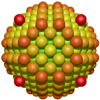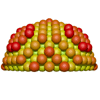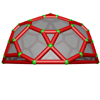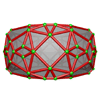# Waterman polyhedra data generator

Written by Paul Bourke
April 2004Root Currently this software supports roots between 1 to 1000 inclusive, should be an integer. Geometry Sphere positions Convex hull Radial cylinders Cones from origin Cones from midway Optionally represent the polyhedra as spheres, cylinders from the origin, or the convex hull of the points. Odd/Even Odd Even The sum of the sphere center coordinates is either even or odd. Origin (0,0,0) Sphere center (1,1,0)/2 Touch point (0,0,1) Octahedron void center (1,1,1)/2 Tetrahedron void center (1,0,1)/2 Origin for distance calculations, default is (0,0,0). Cylinder resolution Low Medium High Cylinders are approximated by planar faces, this option determines the resolution. Low gives coarser cylinders but less geometry. Remove inner spheres No Yes Removes spheres that are either not visible or don't contribute to the convex hull, primarily used to reduced the amount of geometric information for increased performance (Somewhat experimental). Output format Raw OOGL POVRay VRML2 The RAW format provides the most basic geometric data, intended for cases where the data will be manipulated into other formats. OOGL is intended for importing into various existing tools such as those from the Geometry Centre, it may also be usful for manipulation into formats that support a indexed vertex/face list. The POVRay format is obviously for the POVRay raytracer, these files will render without modification. This is the way of creating the highest quality images. VRML will present an interactive 3D model directly within your browser assuming you have a VRML2 plugin. Note that spheres are quite inefficient in VRML so use with care for sphere geometry. Colour map Constant (single colour, grey) blue-cyan-green-magenta-red blue-red Grey scale blue-green-red-green-bluered-blue-cyan-magenta blue-cyan-green-yellow-red-white yellow-red blue-green-red blue-white Apply the colour ramp where appropriate. Rotate X Y Z X Y Z X Y Z Rotate about the axis indicated, angles in degrees. Three separate rotations can be specified, they are applied in the order they are list. Scale Scale the polyhedra by the factor given, the polyhedra is always centered at the origin. Filter <= x <= <= y <= <= z <= Only keep points within the ranges shown, for a polyhedra of root "r" the maximum range is += sqrt(2r).
ExamplesRoot: 24 Spherical geometryRoot: 24 Convex hull geometryRoot: 35 Spherical geometry z >= 0Root: 35 Convex hull geometry z >= 0Root: 66 Convex hull geometry -5 <= z <= 5Root: 110 Convex hull geometry -8 <= y <= 8 z >= 0